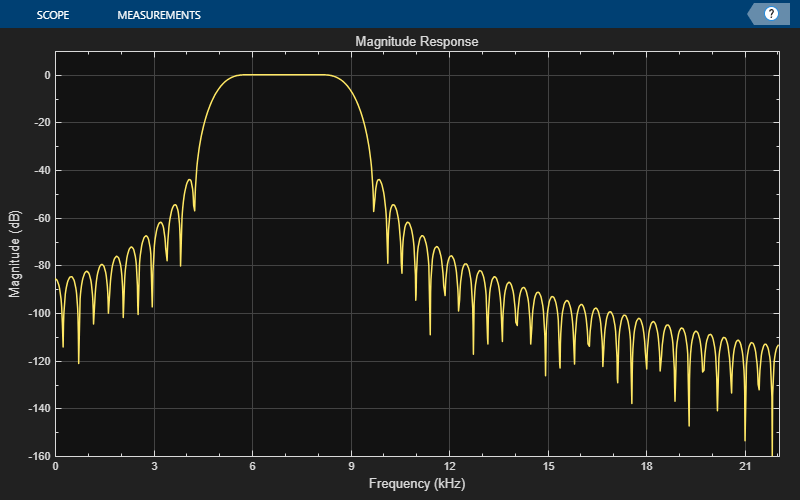# step

Display time-varying magnitude response

## Syntax

``step(dfv,filt)``
``step(dfv,B,A)``

## Description

example

````step(dfv,filt)` displays the time-varying magnitude response of the object filter, `filt`, in the Dynamic Filter Visualizer figure, as long as `filt` has a valid `freqz()` implementation.```

example

````step(dfv,B,A)` displays the magnitude response for the digital filters with numerator and denominator polynomial coefficients stored in `B1` and `A1`, `B2` and `A2`, ..., and `BN` and `AN`, respectively.```

## Examples

collapse all

Design an FIR filter with a time-varying magnitude and phase response. Plot this varying response on a dynamic filter visualizer in normalized frequency units.

Create a `dsp.DynamicFilterVisualizer` object. Set the `PlotAsMagnitudePhase` and the `NormalizedFrequency` properties to `true`.

```dfv = dsp.DynamicFilterVisualizer(PlotAsMagnitudePhase=1,... NormalizedFrequency=true,ShowLegend=true,... Title='Magnitude and Phase Response',... FilterNames="FIR Filter")```
```dfv = dsp.DynamicFilterVisualizer handle with properties: FFTLength: 2048 NormalizedFrequency: 1 FrequencyRange: [0 1] XScale: 'Linear' MagnitudeDisplay: 'Magnitude (dB)' PlotAsMagnitudePhase: 1 PlotType: 'Line' AxesScaling: 'Auto' Show all properties ```

Vary the cutoff frequency of the FIR filter k from `0.1` to `0.5` in increments of `0.001`. View the varying magnitude and phase response using the dynamic filter visualizer.

```for k = 0.1:0.001:0.5 b = designLowpassFIR(FilterOrder=90,CutoffFrequency=k); dfv(b,1); end```Visualize the varying magnitude response of the variable bandwidth FIR filter using the dynamic filter visualizer.

Create a `dsp.DynamicFilterVisualizer` object.

```dfv = dsp.DynamicFilterVisualizer(YLimits=[-160 10],... FilterNames="Variable Bandwidth FIR Filter")```
```dfv = dsp.DynamicFilterVisualizer handle with properties: FFTLength: 2048 NormalizedFrequency: 0 SampleRate: 44100 FrequencyRange: [0 22050] XScale: 'Linear' MagnitudeDisplay: 'Magnitude (dB)' PlotAsMagnitudePhase: 0 PlotType: 'Line' AxesScaling: 'Manual' Show all properties ```

Design a bandpass variable bandwidth FIR filter with a center frequency of 5 kHz and a bandwidth of 4 kHz.

```Fs = 44100; vbw = dsp.VariableBandwidthFIRFilter(FilterType='Bandpass',... FilterOrder=100,... SampleRate=Fs,... CenterFrequency=5e3,... Bandwidth=4e3);```

Vary the center frequency of the filter. Visualize the varying magnitude response of the filter using the `dsp.DynamicFilterVisualizer` object.

```for idx = 1:100 dfv(vbw); vbw.CenterFrequency = vbw.CenterFrequency + 20; end```## Input Arguments

collapse all

Dynamic filter visualizer, specified as a `dsp.DynamicFilterVisualizer` object.

Filter System object with a valid `freqz()` implementation.

Numerator polynomial coefficients, specified as a row vector.

Data Types: `single` | `double`

Denominator polynomial coefficients, specified as a:

• scalar –– The filter is an FIR filter.

• row vector –– The filter is an IIR filter.

Data Types: `single` | `double`

## Version History

Introduced in R2018b